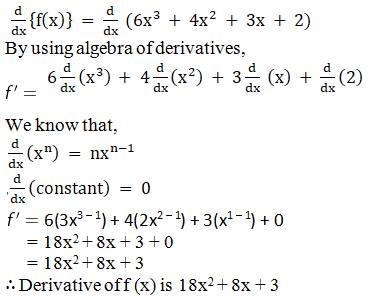# RD Sharma Solutions for Class 11 Chapter 30 - Derivatives Exercise 30.3

Exercise 30.3 mainly deals with fundamental rules for differentiation which includes theorems, with examples the theorems are explained to help students to understand the concepts clearly. Students can solve the exercise wise problems by referring to the solutions to gain a better hold on these concepts. RD Sharma Class 11 Maths Solutions are provided here, where the solutions are formulated by the subject experts to help students gain that extra edge of knowledge. The RD Sharma Solutions are made readily available in the pdf format which can be downloaded easily from the links given below.

## Download the pdf of RD Sharma Solutions for Class 11 Maths Exercise 30.3 Chapter 30 – Derivatives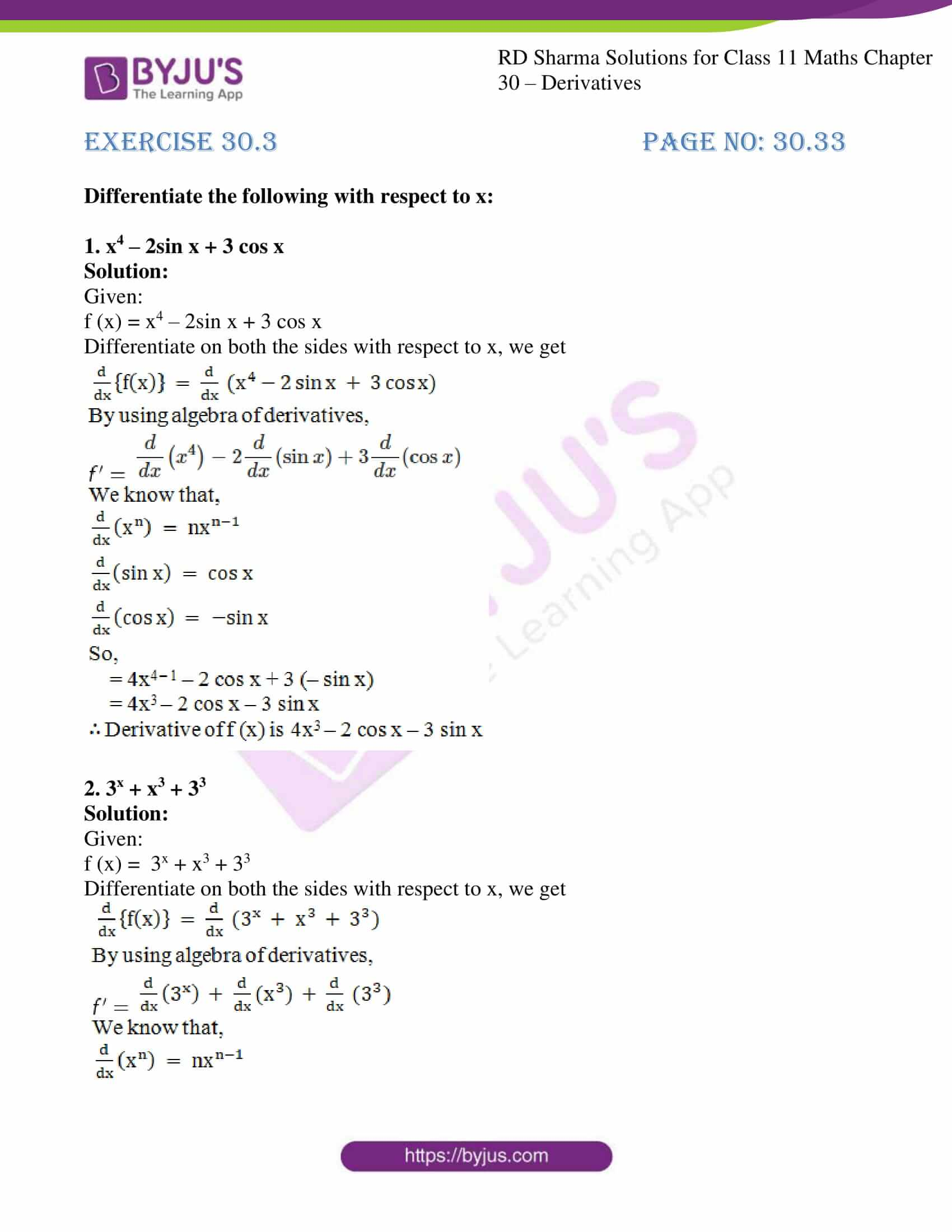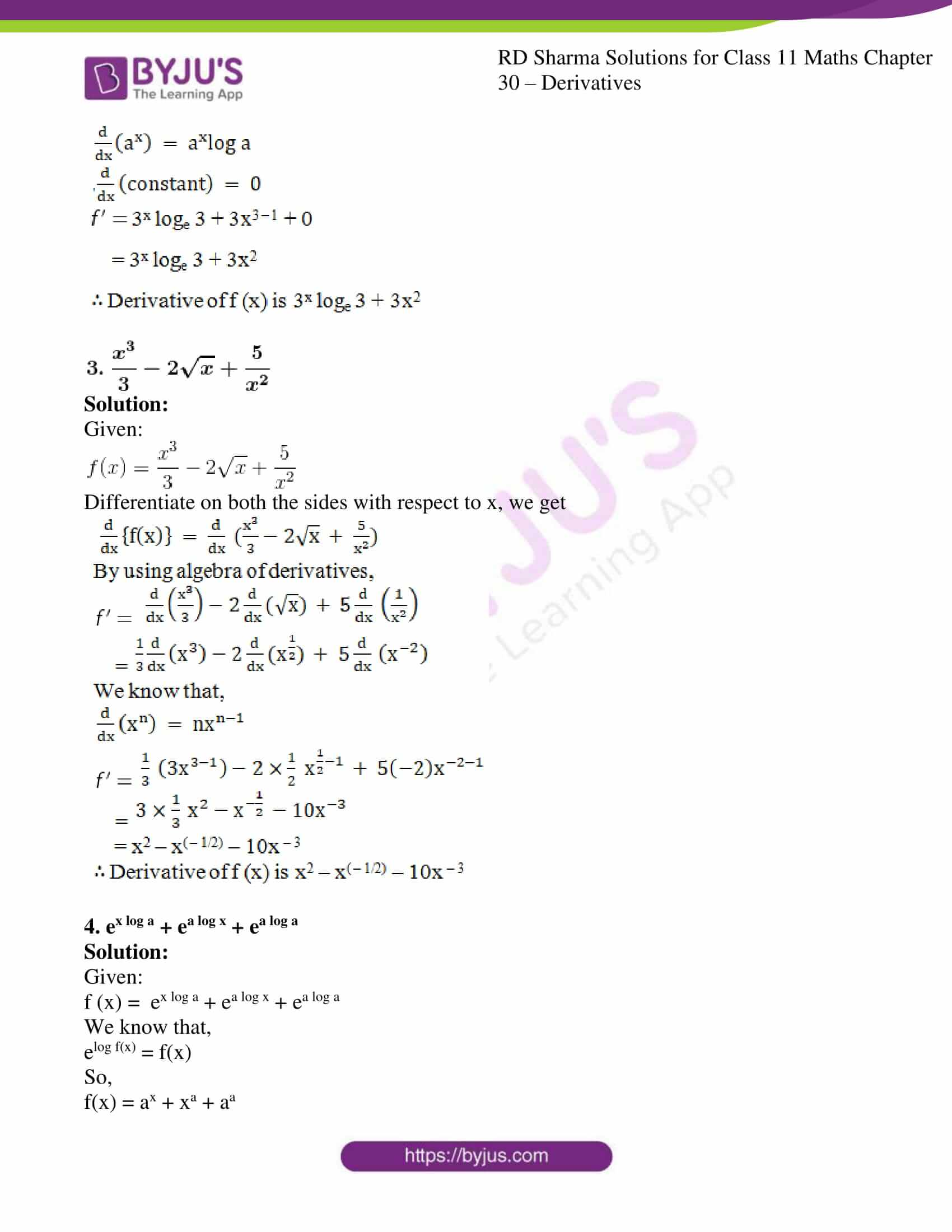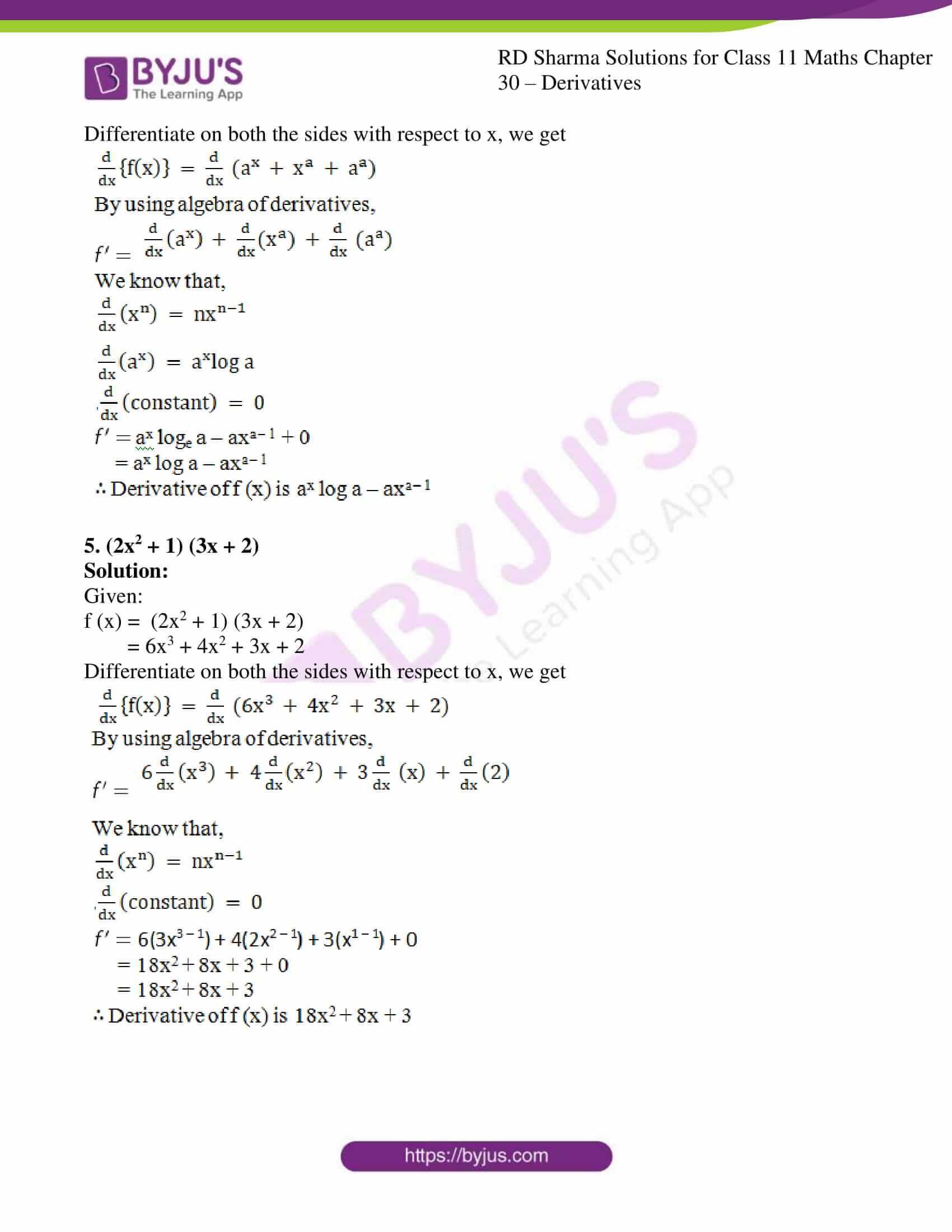### Access answers to RD Sharma Solutions for Class 11 Maths Exercise 30.3 Chapter 30 – Derivatives

#### EXERCISE 30.3 PAGE NO: 30.33

Differentiate the following with respect to x:

1. x4 – 2sin x + 3 cos x

Solution:

Given:

f (x) = x4 – 2sin x + 3 cos x

Differentiate on both the sides with respect to x, we get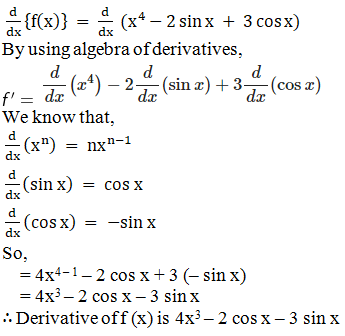2. 3x + x3 + 33

Solution:

Given:

f (x) = 3x + x3 + 33

Differentiate on both the sides with respect to x, we get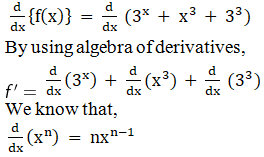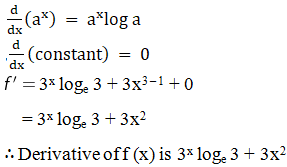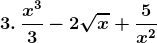Solution:

Given: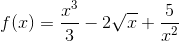Differentiate on both the sides with respect to x, we get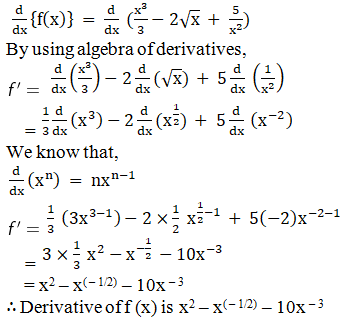4. ex log a + ea log x + ea log a

Solution:

Given:

f (x) = ex log a + ea log x + ea log a

We know that,

elog f(x) = f(x)

So,

f(x) = ax + xa + aa

Differentiate on both the sides with respect to x, we get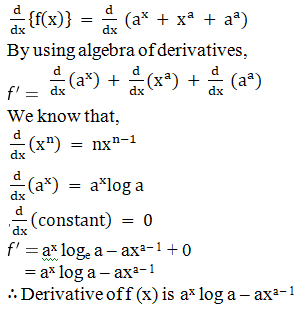5. (2x2 + 1) (3x + 2)

Solution:

Given:

f (x) = (2x2 + 1) (3x + 2)

= 6x3 + 4x2 + 3x + 2

Differentiate on both the sides with respect to x, we get